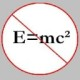You are currently browsing the tag archive for the ‘singleton’ tag.

After postulating a couple of important axioms in the previous two sections, we now arrive at a couple of important results.

1. There exists an empty set. (In fact, there exists exactly one!)

2. The empty set is a subset of every set.

Indeed, to prove the first result, suppose$A$ is some set. Then, the set$\{ x \in A: x \not= x \}$ is clearly an empty set, i.e. it doesn’t contain any elements. To “picture” this, imagine an empty box with nothing inside it. In fact, we can apply the axiom of specification to$A$ with any universally false sentence to create an empty set. The empty set is denoted by$\emptyset$. The axiom of extension, on the other hand, guarantees there can be only one empty set.

Now, how do we argue that$\emptyset \subset A$, for any arbitrary set$A$? Well, the reasoning is an indirect one, and for most beginners, it doesn’t seem like a complete one. There is something in the argument that doesn’t feel quite “right!” However, there is nothing “incomplete” about the argument, and here it is anyway.

Suppose, for the sake of contradiction, the emptyset,$\emptyset$, is not a subset of$A.$ Then, there exists an element in$\emptyset$ that doesn’t belong to$A.$ But, the empty set is empty, and hence, no such element exists! This means our initial hypothesis is false. Hence, we conclude (maybe, still somewhat reluctantly)$\emptyset \subset A$.

Now, the set theory we have developed thus far isn’t a very rich one; after all, we have only showed there is only one set and that it is empty! Can we do better? Can we come up with an axiom that can help us construct new sets? Well, it turns out, there is one.

Axiom of pairing: If$a$ and$b$ are two sets, then there exists a set$A$ such that$a \in A$ and$b \in A$.

The above axiom guarantees that if there are two sets,$a$ and$b$, then there exists another one,$A$, that contains both of these. However,$A$ may contain elements other than$a$ and$b$. So, can we guarantee there is a set that contains exactly$a$ and$b$ and nothing else? Indeed, we can. We just apply the axiom of specification to$A$ with the sentence “$x = a$ or$x = b$.” Thus, the set$\{ x \in A: x = a \mbox{ or } x = b\}$ is the required one.

The above construction of a particular set illustrates one important fact: all the remaining principles of set construction are pseudo-special cases of the axiom of specification. Indeed, if it were given that there exists a set containing some particular elements, then the existence of a set containing exactly those elements (and nothing else) would follow as a special case of the axiom of specification.

Now, observe if$a$ is a set, then the axiom of pairing implies the existence of the set$\{ a, a \}$, which is the same as the set$\{ a \}$ and is called a singleton of$a$. Also, note that$\emptyset$ and$\{ \emptyset \}$ are different sets; the first has no elements at all, whereas the second has exactly one element, viz. the empty set. In fact, there is a minimalist (inductive) way of constructing the set of natural numbers,$N$, (due to von Neumann) using the axiom of infinity as follows.$0 = \emptyset, 1 = \{ 0 \}, 2 = \{ 0, 1 \}, \ldots$

But, more on this later.

### Blog Stats

• 349,162 hitsprof dr drd horia or… on My first postprof drd horia orasa… on My first postprof dr mircea orasa… on Inequality with lognotedscholar on Self-referential Paradoxes, In…prof dr mircea orasa… on Inequality with logprof dr mircea orasa… on Inequality with logprof dr mircea orasa… on 2010 in reviewkenji on Basic category theory, Iprof dr mircea orasa… on Solution to POW-10: Another ha…prof drd horia orasa… on Continued fraction for eprof drd horia orasa… on Inequality with logprof dr mircea orasa… on Solution to POW-12: A graph co…prof dr mircea orasa… on The Character of Physical…prof dr mircea orasa… on Milk and Tea puzzleprof drd horia orasa… on Inequality with log